# Summing Values In a Range Specified By Indirect Cell References in Excel

In this article we will learn about how we can sum the value to a specified range by indirect cell references in Excel.

To sum values in a range specified by indirect cell references in Excel, we will use a combination of SUM & INDIRECT functions to get the output.

SUM: Adds all the numbers in a range of cells

Syntax: =SUM(number1,number2,...)

There can be maximum 255 arguments.Refer below shown screenshot: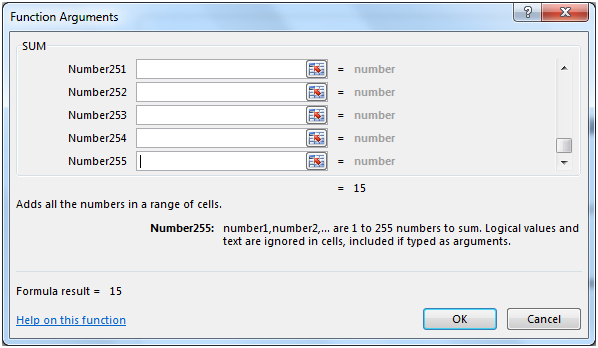INDIRECT: Returns the reference specified by a text string.

Syntax: =INDIRECT(ref_text,A1)

Example: If value in cell A1 contains 10, B1 contains A1 & we use INDIRECT function in cell C1=INDIRECT(B1), then result would be 10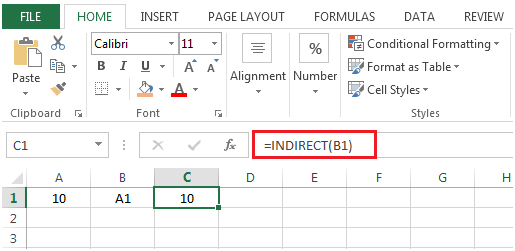Let us take an example:-

Column A contains numbers.Column B contains specified range of cell references that need to be totaled.Cell B1 is the starting range & B2 is the ending range.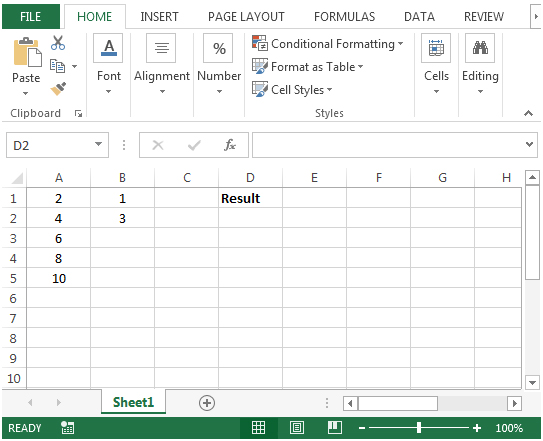• In cell D2, the formula would be
• =SUM(INDIRECT("A"&B1&":A"&B2))
• Press Enter on your keyboard.
• The function will return the total values to range specified by indirect cell reference.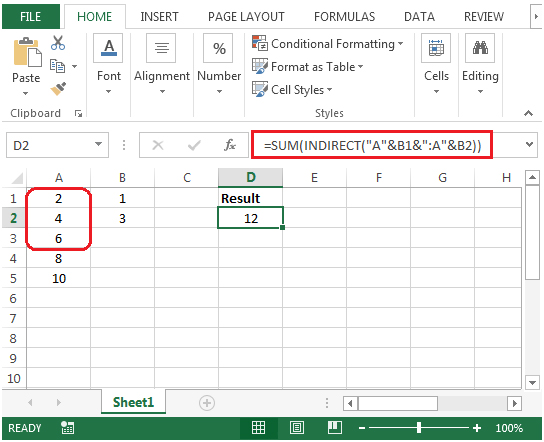The above shown formula will add the sum of values as specified in column B. If we change the value in cell B2 from 3 to 4 then the formula will calculate the result accordingly.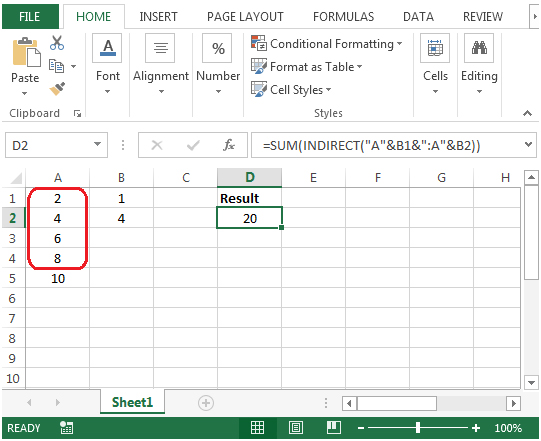1.How to use this formula between 2 sheet.

•If you want to sum from Sheet2 then use this formula.

=SUM(INDIRECT(“Sheet2!A”&B1&”:A”&B2))

where B1 and B2 can have any row number. If B1 has 2 than and B2 has 5 than range sheet2!B2:B5 will be summed up.
You can take help of this article too for summing across different sheet.

https://www.exceltip.com/summing/summing-values-from-cells-in-different-sheets.html

2.Good day,
I tried this formula in a workheet and the result I got was volatile. There are no blanks between the row range. What went wrong please?
Thank you

3.Above shows summing a column. Nice

•If you have data numbers in A1:E1 and column letters (A and E) in A2 and A3 you want to sum in same fashion as above. use this formula
=SUM(INDIRECT(A2&"1:"&A3&"1"))

•How can I change the offset starting reference so that it can change for every row in formula? For example,
E1=SUM(OFFSET(F1,0,0,1,52))
E2=SUM(OFFSET(F2,0,0,1,52))
E3=SUM(OFFSET(F3,0,0,1,52))

•The starting reference will change automatically when you copy down your formula. This is called relative referencing and it is default in Excel Formulas.